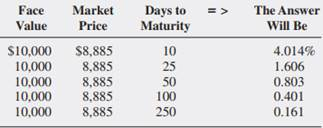### Create an Account

Home / Questions / Using a Spreadsheet to Calculate Tbill Yield What is the quoted yield of a \$10000 face val...

# Using a Spreadsheet to Calculate Tbill Yield What is the quoted yield of a \$10000 face value Tbill with a market price of \$8885 if there are 10 25 50 100 and 250 days to maturity

Using a Spreadsheet to Calculate T-bill Yield: What is the quoted yield of a \$10,000 face value T-bill with a market price of \$8,885 if there are 10, 25, 50, 100, and 250 days to maturity? (LG 5-2)Apr 26 2020 View more View LessSubscribe To Get Solution